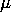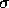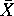# Glossary

alternative hypothesis - the hypothesis that the researcher expects to support.

analysis of variance - a statistical test of the difference of means for two or more groups (also termed ANOVA

ANOVA - ANOVA is an acronym for analysis of variance.  It is a statistical test of the difference of means for two or more groups.

box-plot - Summary plot based on the median, quartiles, and extreme values. The box represents the interquartile range which contains the 50% of values. The whiskers represent the range; they extend from the box to the highest and lowest values, excluding outliers. A line across the box indicates the median.

categorical variable  - a variable that has mutually exclusive ("named") groups that lacks intrinsic order. Major in college and race are examples of a categorical variable.

central tendency - a typical or representative value for a dataset. It can be reported as either the mean, the median, or the mode, depending on the data and/or one's purposes.

Chi Square - a statistical procedure which examines the relationship between two categorical variables. The test is based on the discrepancy between the observed number of observations in each category and the expected number of observations in each category.

coefficient of determination - a statistic used in linear regression that indicates the amount of variation in the dependent variable which is explained or accounted for by the independent variable(s).

confidence interval - the generic label used to describe the decision points where the researcher favors the alternative hypothesis over the null hypothesis. Stated differently, it is the range of mean values within which the true population mean is likely to fall.

continuous variable - a variable which can assume an infinite number of values. Weight is an example of a continuous variable. Between any two measures of weight (e.g., 150 to 151 pounds) lie an infinite number of possible values (e.g., 150.1, 150.2, 150.21, . . .).

convenience sample - this kind of sampling is used when the researcher decides to select the units of study on the basis of their being readily available.

correlation - a standardized index of the strength and direction of the relationship between two variables. The range for the possible correlation between any two variables is from -1.00 (a perfect inverse relationship) to +1.00 (a perfect positive relationship).

covariance - a measure of association between a pair of variables. It is similar to a correlation, but a correlation is expressed in a standardized metric, whereas covariance is expressed in the units of the original variables.

critical value - value that establishes the boundaries of the confidence interval.

decile - a subset of adjacent scores in a distribution representing 10% of a sample or a population. A "decile score" is a raw score corresponding to the 10th, 20th, or 30th etc. percentage score.

degrees of freedom - the number of components in the calculation of a statistic that are free to vary

dichotomous variable - is a discrete measure with two categories that may or may not be ordered.  It is a variable which has only two categories.

discrete variable - a variable which is limited to a finite number of values. A discrete variable usually describes something which occurs only in whole units. The number of males in an English class is an example of a discrete variable.

dispersion - the "spread" of a data set, the departure from central tendency.

distribution - In a distribution, the horizontal axis (x-axis) represents the variable being described. The density of the smooth curve over the x-axis represents the probability of occurrence for each of the values on the x-axis.

explained variance - the variance in Y about Y' where Y' is the value of Y on the regression line predicted by the regression equation. If the regression line does not help in predicting Y, then it will pass through Y-bar, in which case, Byx= 0.  In absolute value terms, the highest possible score for Byx= +/- 1.00.

heteroscedasticity - a condition in which the variances of two or more population distributions are not equal.

histogram - a bargraph used to represent the frequency of each value occurring in a distribution of scores.

homoscedasticity - a condition in which the variances of two or more population distributions are equal.

hypotheses - a set of two or more mutually exclusive and often exhaustive statements. The goal of hypothesis testing is to determine which is true.

independent samples t-test - In hypothesis testing, this is the procedure used to compare the means of two different samples. As is true for all t-tests, the standard error is not known and is estimated from sample data.

interval data - data that possess magnitude (one value can be judged greater than, less than, or equal to another) and a constant distance between intervals (units of measurement are the same on the scale regardless of where the unit falls). Temperature is an example of interval data: the difference between 100 degrees and 99 degrees is the same as the difference between 40 degrees and 39 degrees. Interval data do not necessarily have an absolute zero point (i.e., a temperature of zero degrees does not indicate that there is no temperature).

interval variable - is a variable whose attributes are rank ordered and have equal distances between adjacent attributes.  An example of an interval variable would be the Fahrenheit temperature scale.

kurtosis - the degree of flatness or peakedness of a graph of a frequency distribution. The relatively flat distributions are described as platykurtic. Distributions with medium curvature are mesokurtic (note: a normal distribution is mesokurtic).  The most peaked distributions are leptokurtic.

leptokurtic - a distribution that is more peaked than a normal distribution.  This is to say there are more cases concentrated close to the mean than in a normal distribution.

line of best fit (least squares fit)  the least squares fit procedure allows us to reduce the scatterplot to a single straight line described by a linear equation. It minimizes the square of the vertical distance between each point and the regression line.

marginal - the frequency distribution of each of two crosstabulated variables. There are row marginals and column marginals.

mean - a measure of central tendency calculated by dividing the sum of the scores in a distribution by the number of scores in the distribution. This value best reflects the typical score of a data set when there are few outliers and/or the dataset is generally symmetrical.

median - the value in a data set which divides the scores into two equal halves (i.e., an equal number of scores lie above and below it). As a measure of central tendency, it is largely unaffected by extreme values.

mode - the score that occurs most frequently in a data set. This measure of central tendency is the only one appropriate for nominal data.

negative skew - asymmetry in a distribution in which the scores are bunched to the right side of the center. With a negatively skewed distribution, the mean generally falls to the left of the median and the median usually lies to the left of the mode. Study Hint: the tail of a negatively skewed distribution points to the negative side of the number line.

nonprobability sample - a type of sampling that involves the researcher's judgment to determine the elements to be selected for the sample.

nominal data - data that are classified into mutually exclusive ("named") groups that lack intrinsic order. Major in college and race are examples of nominal data.

normal distribution - a theoretical distribution which is typically bell-shaped when graphed. The distribution is theoretical because the height of the curve is defined by a mathematical formula (and the exact values necessary to create the curve would never occur).

null hypothesis - the prediction that the researcher believes will be "nullified." That is, the researcher believes this prediction is not true.

observation - the empirical data that it used to support or refute a hypothesis

ordinal data - data whose values are ordered so that we can make inferences regarding magnitude, but which have no fixed interval between values. An example of ordinal data is a letter grade on a test.

ordinal variable - is a variable whose values are ordered so that we can make inferences regarding magnitude, but which have no fixed interval between values. Letter grade on a test would be an ordinal variable: while an 'A' is greater than a 'B' which is greater than a 'C', we cannot conclude that the distance between an 'A' and a 'B' is the same as the distance between a 'B' and a 'C'.

outlier - a value in a data set that is very different from most other values in the set.

paired t-test - In hypothesis testing, this is the procedure used when the independent variable is within subjects in nature. The goal is to compare two levels of the independent variable assigned to the same group of subjects at different points in time. As is true for all t-tests, the standard error is not known and is estimated from sample data.

parameter - a characteristic of a population, e.g. mean (), pronounced "myu", and standard deviation (), or "sigma".

pearson's correlation coefficient - a measure of association between two continuous variables which estimates both the direction and strength of a linear relationship.

percentile - A value that exceeds a specific percentage of the distribution. Thus, if the 63rd percentile score for a set of students on the SAT verbal exam is 560, then 63% of scores are at or below 560.

platykurtic - a distribution that is flatter than a normal distribution.  This is to say that there are more cases in the tails of the distribution than in a normal distribution.

population - the set of all possible data values that could be observed.

positive skew - asymmetry in a distribution in which the scores are bunched to the left side of the center. With a positively-skewed distribution, the mean generally falls to the right of the median and the median usually lies to the right of the mode. Study Hint: the tail of a positively skewed distribution points to the positive side of a number line.

probability sample - sampling in which each element within a study population has a known, nonzero chance of being selected into the sample.

protocol - a specified methodology for performing a task

quartile - a subset of adjacent scores in a distribution representing 25% of a sample or a population. A "quartile score" is a raw score corresponding to the 25th, 50th, or 75th percentile score.

quintile - A subset of adjacent scores in a distribution representing 20% of a sample or a population. A "quintile score" is a raw score corresponding to the 20th, 40th, 60th, or 80th percentile score.

random sample - a sample that contains observations which are selected form a population so that every member of the population has a known chance of selection for a sample.

random variable - the measurements of a random variable vary in a seemingly random and unpredictable manner.  A random variable assumes a unique numerical value for each of the outcomes in the sample space of the probability experiment.

range - a simple measure of dispersion, indicating the difference between the lowest and highest values observed.

ranked categories - categories within a variable that are logically ranked.  The different attributes of each category represent relatively more or less of the variable.

ratio data - data that are ordered (so that we can make inferences regarding magnitude), have equal intervals between values, and contain an absolute zero point. Height is an example of ratio data: 60 inches is taller than 55 inches, the distance between 60 and 55 inches is the same as the distance between 30 and 25 inches, and a height of 0 inches implies no height at all.

ratio variable - these are variables that are based on a true zero point.  An example of a ratio variable would be age.

regression - a statistical procedure that allows us to determine the extent to which we can predict a given observation's score on a dependent variable, given that observation's score on one or more independent variables.

regression coefficient - the slope of the regression line.  It represents the change in y for every one unit change in x.

regression line - a model that simplifies the relationship between two variables.  By approximating a line through the center of a scatterplot that represents the data, we create a two dimensional center for the data.  The line summarizes the data points in the same way that measures of central tendency do.

sample - a collection of observations selected form a larger population.

sampling distribution - all possible non-overlapping samples that can be drawn, given a constant sample size.

sampling distribution of means - a frequency distribution of a large number of random sample means that have been drawn from the same population.

sampling distribution of the difference between means - a sampling distribution that consists of the differences in means between groups.

sampling distribution of means - a frequency distribution of a large number of random sample means that have been drawn from the same population.

sampling distribution of the mean of difference scores - a sampling distribution that consists of the differences in means within subjects across treatments.

sampling error - the extent to which a sample distribution is different than the population distribution from which the sample is drawn.

scatterplot - a group of data points that are plotted along x-axis and y-axis coordinates. Every individual is represented as a data point, whereby a perpendicular line from the individual's "X" value intersects a perpendicular line from the individual's "Y" value.

single sample t-test - In hypothesis testing, this is the procedure used to compare the mean of one sample to a known population mean. As is true for all t-tests, the standard error is not known and is estimated from sample data.

skewness - asymmetry in a distribution in which scores are bunched on one side of the distribution. See positive skew, negative skew.

standard deviation - a measure of dispersion describing the spread of scores around the mean. It is the square root of the variance.

standard error - the standard deviation of a sampling distribution.

standard error of the mean - the standard deviation of a sampling distribution of means.

standard error of the mean of difference scores - the standard deviation of a sampling distribution of the mean of difference scores.

standard score - a raw score that has been converted from one scale into another scale with an arbitrarily set mean and standard deviation. Standard scores are more easily interpreted than raw scores, because they take into account the mean and standard deviation of the distribution of values.

statistic - a characteristic of a sample, e.g. mean () and standard deviation(s).

strata - a subdivision of a population.

stratification - allocating samples among subcategories, called strata, within a population. Stratification is sometimes necessary to improve the effectiveness of a sampling effort or to increase understanding of population characteristics. For example, stratifying an election survey by sex allows analysts to better understand voter behavior by revealing differences in the way that males and females vote.

type I error - erroneously rejecting the null hypothesis: concluding that a sample came from a different population when it in fact is from the same population.

type II error - erroneously failing to reject the null hypothesis: concluding that a sample came from the given population when it in fact is from a different population.

variance - a measure of dispersion, indicating the mean of the squared deviations of a set of scores from the mean of the scores.

y-intercept - the point through which the line intersects the Y-axis.  It is the value of y when x equals zero.

z score - a standardized score which indicates the how many standard deviations a value lies above or below the mean.

## Study Hint for Remembering the Types of Data

The combined first letters of each type spell NOIR, which is the French word for black.

Updated August 2, 1999